Technical Article

# Analyzing Circuits via Source Transformation

November 01, 2016 by Sneha H.L.

## This article uses examples to explain the technique of source transformation.

### Background Study

An electrical network can consist of sources and passive elements. Sources are circuit components which possess their own energy and are capable of transferring this energy to other circuit elements.

There are two basic types of sources: voltage sources and current sources. These can be further classified as independent or dependent. In the case of independent sources, the voltage or current is fixed. If the source is dependent, the value of the voltage or current depends on the amount of current or voltage elsewhere in the circuit.

Passive components do not have their own energy. As a result of this, they are regarded as sinks. However, they influence the amount of current or voltage in a given portion of the circuit. Resistors, capacitors, and inductors are passive components.

### Analyzing Electrical Networks

The complexity of electrical networks ranges from very simple—e.g., a voltage divider—to very complicated—e.g., the internal structure of an integrated circuit (IC).

A good electrical designer is expected to have a sound knowledge of the entire system irrespective of its complexity. This is absolutely essential when the issue arises of upgrading or troubleshooting the system.

At this point, it is essential to note that analyzing an electrical circuit is sometimes easy and straightforward, taking only a couple of minutes in its entirety. Sometimes, though, it can involve a lot of hard work (or rather, smart work), and it may even force the analyzer to resort to help from software. Nevertheless, the mode of analysis is based on certain basic rules and theorems.

Here's a list of important theorems along with a brief explanation:

1. Superposition Theorem: Aids in finding the current and voltage in a circuit which has multiple sources; the effects produced by each of the sources individually can be summed.
2. Thevenin's Theorem: Aids in circuit simplification; multiple sources and resistances can be represented by an equivalent circuit with just a single voltage source and a single resistor.
3. Norton's Theorem: Aids in circuit simplification; multiple sources and resistances can be represented by an equivalent circuit with just a single current source and a single resistor.
4. Millman's Theorem: A simplification technique involving circuits with parallel branches.

At this point, it should be noted that all these theorems are based on the basic rules governing the field of electronics, namely, Ohm's Law and Kirchoff's Laws.

In addition, we may sometimes find a circuit which has the resistors connected either in a delta/pi or a star/Y/T configuration. In such cases, we can employ star-to-delta or delta-to-star transformation when analyzing the circuit.

### Source Transformation for Independent Sources

Consider the circuit shown in Figure 1; the goal is to find the current (denoted by i) through the central 5 Ω resistor. Here, mesh analysis (Kirchoff's Voltage Law, KVL) cannot be applied readily because the circuit has a branch which has a current source. Thus, we need to devise a method by which we can eliminate this current source from our circuit. However, while doing so, we need to take care that the current and the voltages in the circuit remain unaltered.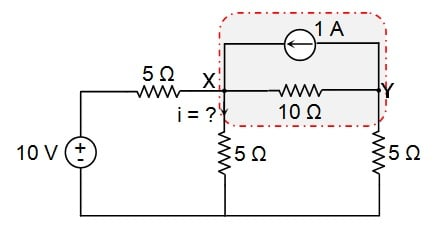##### Figure 1

Recall Ohm's Law, which states that $$I = \frac{V}{R}$$.

#### Step 1: Current-to-Voltage Source Transformation

Looking back at the circuit (Figure 1) again, we can see that the 1 A current source has a 10 Ω resistor in parallel with it. Let us now replace this combination with a voltage source, V = 1 A × 10 Ω = 10 V, and a 10 Ω series resistor. You can see what this looks like in Figure 2. Note that the positive terminal of the voltage source is placed to the left, because the current-source arrow was pointing to the left. These two circuits (Figure 1 and Figure 2) are considered to be equivalent: the 1 A current entering node X from node Y has not changed.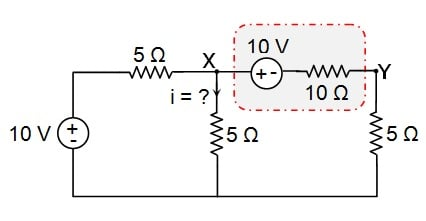##### Figure 2

The process carried out here is called source transformation. We have transformed an existing current source with a parallel resistor into an equivalent voltage source with a series resistor.

#### Step 2: Voltage-to-Current Source Transformation

The circuit in Figure 2 can be further simplified as it has a 10 Ω resistor in series with a 5 Ω resistor. These can be replaced by an equivalent 15 Ω (= 10 Ω + 5 Ω) resistor. The simplified circuit is shown in Figure 3(a).

Now we can easily apply mesh analysis to solve the problem before us. However, there is also a much easier graphical way of achieving this: apply source transformation again!

Previously we transformed a current source with a parallel resistor, but we can also apply source transformation to a voltage source with a series resistor. We have two such arrangements, as indicated in Figure 3(b). This circuit is equivalent to the one shown in Figure 3(a).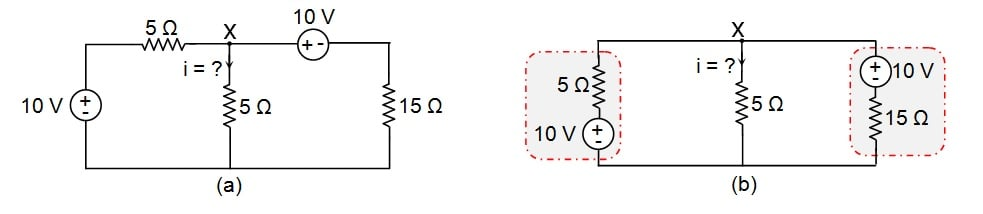##### Figure 3

So here we will apply voltage-to-current source transformation, which is very similar to current-to-voltage source transformation. The process involves replacing the voltage source V in series with a resistor R with an equivalent network which has a current source $$I = \frac{V}{R}$$ in parallel with a resistor R. The current source is oriented such that the arrow points towards the positive terminal of the voltage source being replaced (see Figure 4).

Thus, for the leftmost branch, we have a current source $$I = \frac{10}{5} = 2\ A$$ in parallel with a 5 Ω resistor. Similarly, for the rightmost branch, we get $$I = \frac{10}{15} = \frac{2}{3}\ A$$ in parallel with a 15 Ω resistor. The resulting circuit is shown in Figure 4.

The circuit in Figure 4 has two current sources pointing in the same direction, and hence these can be replaced by a single current source whose value is equal to their sum, i.e., $$\frac{8}{3}\ A$$.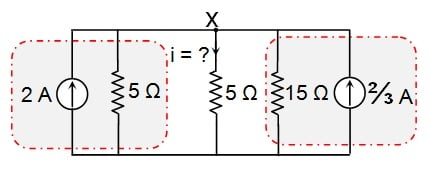##### Figure 4

There are three resistors: two 5 Ω resistors and one 15 Ω resistor, all in parallel. We could replace all three of them with an equivalent resistance (REQ), but our goal is to find the current through the 5 Ω resistor, so we will combine only the other two.

$$R_{EQ} = \frac{5 × 15}{5 + 15} = \frac{5 × 15}{20} = \frac{15}{4}\ \Omega$$

Having made these changes, we get the circuit shown in Figure 5.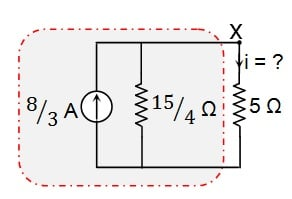#### Step 3: Current-to-Voltage Source Transformation (again)

Now, let us apply current-to-voltage source transformation once again for the combination indicated in Figure 5.

Here, the voltage source will be of the value $$V=\frac{8}{3}\times\frac{15}{4}=10\ V$$, with the positive terminal towards node X, in series with a $$\frac{15}{4}\ \Omega$$ resistor.

The resulting circuit is shown in Figure 6.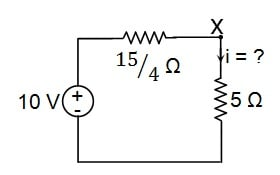##### Figure 6

For Figure 6, we can readily apply KVL to obtain the current through the 5 Ω resistor:

$$10 - \frac{15}{4}i - 5i = 0$$

$$10 - \frac{35}{4}i = 0$$

$$10 = \frac{35}{4}i$$

$$i = 10\times\frac{4}{35} = \frac{8}{7}\ A$$

### Source Transformation for Dependent Sources

Source transformation is applicable even for the circuits which have dependent sources. Let's consider the circuit shown in Figure 7(a).

Here, one needs to resort to source shifting for the 3 A current source before applying source transformation. This yields the circuit shown in Figure 7(b).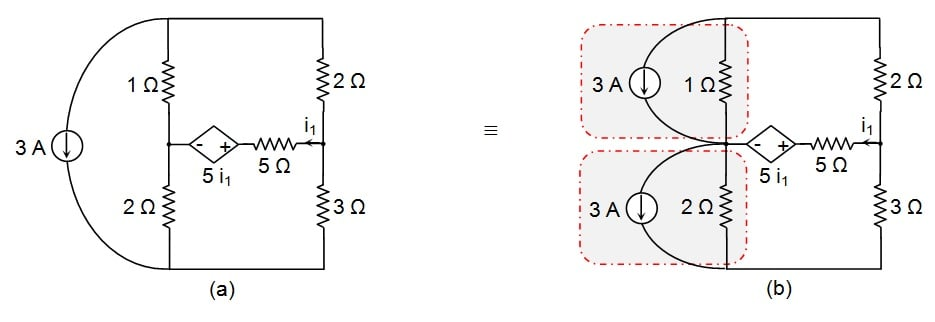#### Step 1: Current-to-Voltage Source Transformation

Now let us apply current-to-voltage source transformation for the circuit elements indicated in Figure 7(b).

For the upper portion, we have V = 3 × 1 = 3 V, with the positive terminal oriented downwards, in series with a 1 Ω resistor. Similarly, for the lower portion we get V = 3 × 2 = 6 V, oriented downwards, in series with a 2 Ω resistor. This leads to the circuit shown in Figure 8(a).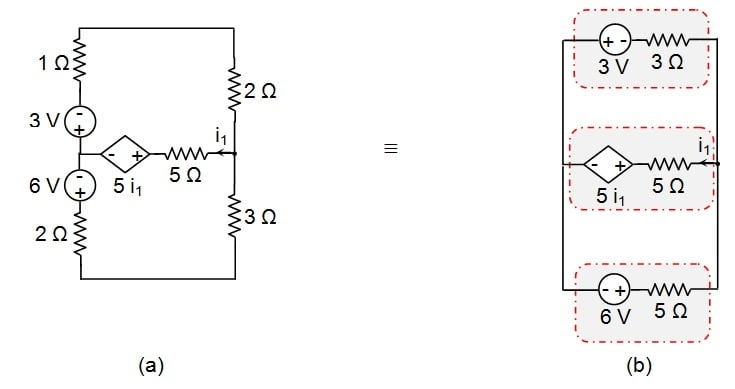##### Figure 8

The circuit can be further reduced: the upper mesh has a 1 Ω resistor in series with a 2 Ω resistor, forming an equivalent resistance of 3 Ω, and the lower mesh has a 2 Ω resistor in series with a 3 Ω resistor, which can be replaced by a single 5 Ω resistor. This results in the circuit shown in Figure 8(b).

#### Step 2: Voltage-to-Current Source Transformation

By looking at Figure 8(b), you can see that we need to apply voltage-to-current source transformation three times (once for each source-plus-resistor combination).

Case 1: For the 3 V source in series with 3 Ω

$$I = \frac{3}{3}=1\ A$$ in parallel with a 3 Ω resistor, directed towards the left.

Case 2: For the 5i1 dependent voltage source in series with 5 Ω

$$I =\frac{5i_{1}}{5}= 1i_{1}\ A$$ in parallel with a 5 Ω resistor, directed towards the right.

Case 3: For the 6 V source in series with 5 Ω

$$I = \frac{6}{5}\ A$$ in parallel with a 5 Ω resistor directed towards the right.

These steps lead to the circuit shown in Figure 9.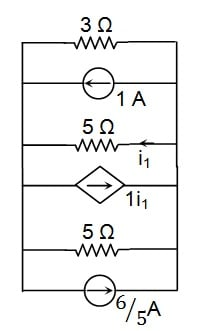##### Figure 9

Here, the two independent current sources are oriented in opposite directions and thus can be replaced by a single current source whose value is given by $$I = \frac{6}{5}-1 = \frac{1}{5}\ A$$, directed towards the right.

Next, we can express the value of the dependent current source as $$I = \frac{1}{5}+1i_{1} = \frac{1+5i_{1}}{5}\ A$$, towards the right.

We need to find the current flowing through the 5 Ω resistor (the one in the middle). Therefore, we will leave it as it is and replace the other two (i.e., the 3 Ω and 5 Ω resistors on top and bottom) with an equivalent resistance: R = 5 || 3 = $$\frac{5\times 3}{5+3} = \frac{15}{8}\ \Omega$$.

The circuit can now be depicted as follows: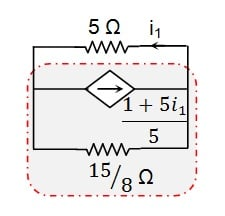#### Step 3: Current-to-Voltage Source Transformation (again)

Finally, we can transform the dependent current source into a dependent voltage source with the value

$$V' = \frac{1+5i_1 }{5} × \frac{15}{8} = \frac{3+15i_1}{8}\ V$$

This will be in series with a $$\frac{15}{8}\ \Omega$$ resistor and will have its positive terminal towards the right.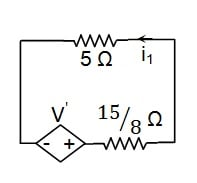##### Figure 11

Now, KVL can be used to compute i1:

$$\frac{3+15i_1}{8}-\frac{15}{8}\times i_1-5i_1 = 0$$

$$\frac{3}{8}+\frac{15i_1}{8}-\frac{15}{8}\times i_1-5i_1 = 0$$

$$\frac{3}{8}-5i_1 = 0$$

$$\frac{3}{8} = 5i_1$$

$$i_1 = \frac{3}{40}\ A$$

### Source Transformation for Circuits with Inductors and Capacitors

Note that source transformation is also applicable for circuits which have inductors and capacitors. However, in this scenario, one needs to analyze the circuit in the frequency domain.

Let's look at the circuit shown in Figure 12(a).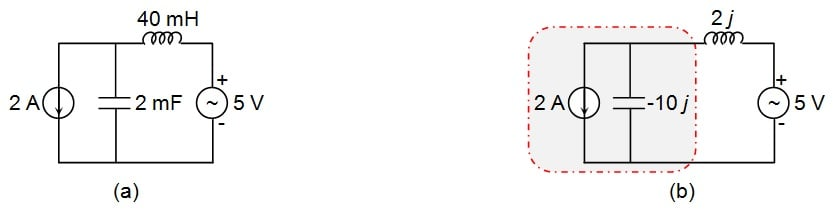##### Figure 12

Here, if we assume ω to be 50 rad/s, then

• Impedance of 2 mF capacitor = -j/ωC = -j/(50 × 2 × 10-3) = -j/(100 × 10-3) = -10j
• Impedance of 40 mH inductor = jωL = j × 50 × 40 × 10-3 = 2j

Now, let us assume that we need to find the voltage across the 40 mH inductor.

When we look at the circuit diagram, it is evident that this process will become easier if we convert the 2 A current source in parallel with a -10j impedance into a voltage source. This process yields V = 2 × (-10j) = -20j directed downwards, in series with a -10j impedance.

The resulting circuit is shown in Figure 13.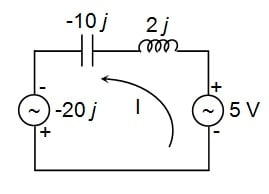##### Figure 13

Now, let us apply KVL to the circuit to determine the current I flowing through it.

$$5-2jI + 10jI-20j = 0$$

$$\left(10j-2j\right)I = 20j-5$$

$$8jI = 20j-5$$

$$I = \frac{20j-5}{8j} = \left(2.5+0.625j\right)\ A$$

Thus, the voltage across the inductor VL will be

$$5-V_L + 10jI-20j = 0$$

$$5-V_L + (10j)\times(2.5+0.625j)-20j = 0$$

$$5 + \left(-6.25+25j\right)-20j = V_L$$

$$V_L = \left(-1.25+5j\right)\ V$$

### Conclusion

The analysis presented in this article on source transformation can be summarized in the following three points:

1. Source transformation carried out per the rules shown in Figure 14 can be used to simplify circuits and facilitate mesh analysis.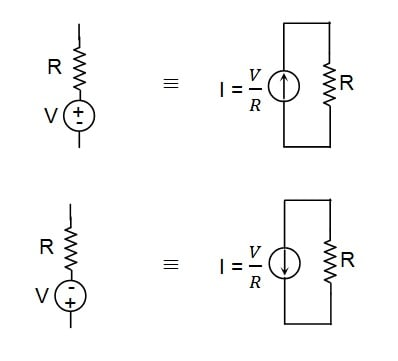##### Figure 14

2. Dependent sources can be transformed just like independent sources (Figure 15).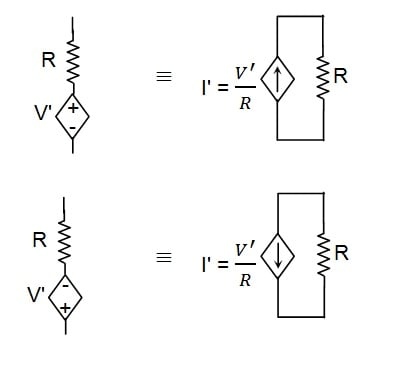##### Figure 15

3. The technique of source transformation can even be used to analyze circuits which have capacitors and inductors, provided we analyze them in the frequency domain.

I hope this article has given you a better understanding of source transformation.

• Share•Bach Nguyen November 03, 2016

Can you please explain more about the source shifting in Fig 7? I can understand that since 3[A] is both entering and leaving the center node, it has zero effect on the KCL at that node. But I haven’t learn about the rule for source shifting yet and Google just come back to this article, so is there any rule for shifting current source/voltage source?

Like.
•snehahl November 10, 2016
The source shifting in Figure 7 is carried out with an intention to aid the process of source transformation i.e. to get a parallel combination of a current source and a resistor. However while doing so, we should ensure that the current through the nodes of the network remains unchanged. This forms the basic rule of source shifting process. Further, we should also take care of polarities of the sources being shifted, which can be considered to be the second rule. The same rules hold good even while shifting the voltage sources.
Like.
•benallgor November 04, 2016

Very good article.  I pride myself on my circuit analyses, but I have rarely used any of these transformations.  In fact I had sit here and prove the source shifting to myself.  I am adding source transformation to my tool box.  I can see that, in many cases it would simplify things.

Like.
•snehahl November 07, 2016
Thank you.
Like.
• R

Very Informative Article ,.. very clearly explained. Thanks so much !!

Like.
• B
ba38 February 17, 2017

Hi all
Nice demo.
BUT the equivalence of the voltage source current dependent “5i1” and resistor 5 ohm in figure 9 explained in the “case 2” is simply FALSE !

Please look at the schema :
http://electronics.stackexchange.com/questions/84816/source-transformation-of-dependent-sources

To have a TRUE equivalence between two branches either the voltage AND the current at the end nodes of the branch MUST be the same.

This is not the case here.

In fact it is not necessary to make an equivalence of the above branch. Make rather voltage source equivalence of the two 1A and 6/5A current sources.

The I1 current is 3/95 A.

I play with LTSpice simulator (operating point) and obviously find this current value (0.031579 A).

Best regards.
Bernard

Like.
• L
Luke53 June 29, 2017

Hi, I agree with the previous remark, I have used “Circuit 4” on my TI89 calculator to solve this and this gives i1 =0.0315 A, same result as Ba found, I am not so sure if one can split-up te 3 A current source into two current sources each in series with the resistors, taking into account that there is a branch between the two resistors, and working out like this gives the result that i1 = 0 and this is not the case here. So I think the mistake is in splitting up the 3 A current source in series with the resistors, witch is in my view not allowed in this case.
Grts.
Luc.

Like.
•RK37 April 23, 2019
My simulations indicate that the two versions of the circuit in Figure 7 are indeed mathematically equivalent, but I agree with your value for i1: 31.6 mA instead of 75 mA. I'm wondering if the error is not in the author's overall approach but rather in the math.
Like.
•David Jide April 06, 2018

Thank you. This was so easy to understand.

Like.
•snehahl April 06, 2018
Thanks.
Like.
• P
pscollard April 19, 2019

Using Figure 13 when converting from Rectangular to Polar and then back to Rectangular the equation is as follows: (20j-5) / 8j = (20.6 < -14.04) / (8 < 0) When I do the division and convert back I get 2.5j - 0.625, not + 0.625. Which is correct? Thanks!

Like.
•RK37 April 23, 2019
If the rectangular form is 8j, the angle in the polar form should be 90°, not 0°.
Like.